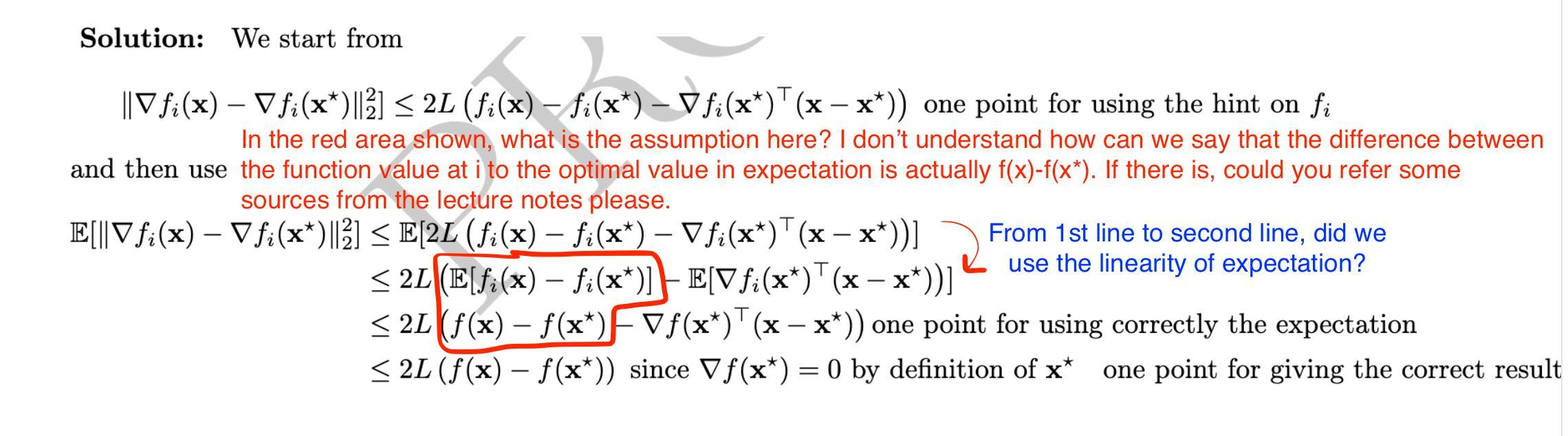Q23 Exam 2020

Hello,
Anyone has understood how we derived the part shown in the image of the solution below?Thx

Top comment

Note that the expectation is taken with respect to the randomness i which is a uniform distribution over {1,...,n}. So the expectation of $$f_i$$ will be $$f$$.

Blue parts: Yes, we use the linearity of expectation.

Page 1 of 1